# Planimetrics - math word problems

Study plane measurements, including angles, distances, and areas. In other words - measurement and calculation of shapes in the plane. Perimeter and area of plane shapes.

#### Number of problems found: 1973

• A large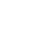A large gear will be used to turn a smaller gear. The large gear will make 75 revolutions per minute. The smaller gear must make 384 revolutions per minute. Find the smallest number of teeth each gear could have. [Hint: Use either GCF or LCM. ]
• Parcelparcel has a rectangular shape of a trapezoid with bases 12 m and 10 m and a height 8 m. On parcel was built object with a footprint an isosceles triangle shape with side 4 m and height three-quarters of a meter. What is the area of unbuild parcel?
• PlotThe plot on which Mr. Kalous is to build a house has the shape of a rectangle. On a 1: 500 scale, its dimensions are 7cm and 5.5cm. Find out the dimensions of the plot. Calculate the parcel size.
• Three points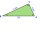Three points K (-3; 2), L (-1; 4), M (3, -4) are given. Find out: (a) whether the triangle KLM is right b) calculate the length of the line to the k side c) write the coordinates of the vector LM d) write the directional form of the KM side e) write the d
• BearingA plane flew 50 km on a bearing 63°20' and the flew on a bearing 153°20' for 140km. Find the distance between the starting point and the ending point.
• Cone A2VThe surface of the cone in the plane is a circular arc with central angle of 126° and area 415 cm2. Calculate the volume of a cone.Calculate the surface area and volume of a regular quadrangular pyramid: sides of bases (bottom, top): a1 = 18 cm, a2 = 6cm angle α = 60 ° (Angle α is the angle between the sidewall and the base plane.) S =? , V =?
• PrismCalculate the volume of the rhombic prism. The prism base is a rhombus whose one diagonal is 47 cm, and the edge of the base is 28 cm. The edge length of the base of the prism and height is 3:5.
• Angles of elevationFrom points A and B on level ground, the angles of elevation of the top of a building are 25° and 37° respectively. If |AB| = 57m, calculate, to the nearest meter, the distances of the top of the building from A and B if they are both on the same side of
• Triangles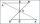Hanka cut the 20 cm long straws into three pieces each piece had a length in cm. Then, with these three pieces, she tried to make a triangle. a) What circuit has each of the triangles? b) How long can the longest side measure? c) How many different triang
• Two parallel chordsIn a circle 70 cm in diameter, two parallel chords are drawn so that the center of the circle lies between the chords. Calculate the distance of these chords if one of them is 42 cm long and the second 56 cm.
• Rectangular gardenRectangular garden has a length of 48.7 m, a width of 6.3 meters shorter than the length. How much mesh should be bought for its fencing if the gate is 2.9 m long and the gate 1.1 m? What is the area of the garden?
• The spacecraft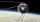The spacecraft spotted a radar device at altitude angle alpha = 34 degrees 37 minutes and had a distance of u = 615km from Earth's observation point. Calculate the distance d of the spacecraft from Earth at the moment of observation. Earth is considered a
• Scale of planOn the plan of the village in the scale of 1: 1000 a rectangular garden is drawn. Its dimensions on the plan are 25mm and 28mm. Determine the area of the garden in ares.
• Sidewalk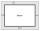In the garden, a 1-meter-wide sidewalk will be paved with tiles around the block-shaped pool. In the picture, the sidewalk is shown in gray. The dimensions of the bottom of the pool are 8.5 meters and 6 meters. The height of the pool walls is 2 meters. Ho
• Two chordsIn a circle with radius r = 26 cm two parallel chords are drawn. One chord has a length t1 = 48 cm and the second has a length t2 = 20 cm, with the center lying between them. Calculate the distance of two chords.
• Base of prismThe base of the perpendicular prism is a rectangular triangle whose legs length are at a 3: 4 ratio. The height of the prism is 2cm smaller than the larger base leg. Determine the volume of the prism if its surface is 468 cm2.
• Segment in a triangle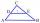In a triangle ABC with the side/AB/ = 24 cm is constructed middle segment/DE/ = 18 cm parallel to the side AB at a distance 1 cm from AB. Calculate the height of the triangle ABC to side AB.
• Pine woodFrom a trunk of pine 6m long and 35 cm in diameter with a carved beam with a cross-section in the shape of a square so that the square had the greatest content area. Calculate the length of the sides of a square. Calculate the volume in cubic meters of lu
• Tank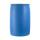In the middle of a cylindrical tank with a bottom diameter 251 cm is standing rod which is 13 cm above the water surface. If we bank rod its end reach surface of the water just by the tank wall. How deep is the tank?

Do you have an interesting mathematical word problem that you can't solve it? Submit a math problem, and we can try to solve it.

We will send a solution to your e-mail address. Solved examples are also published here. Please enter the e-mail correctly and check whether you don't have a full mailbox.

Please do not submit problems from current active competitions such as Mathematical Olympiad, correspondence seminars etc...